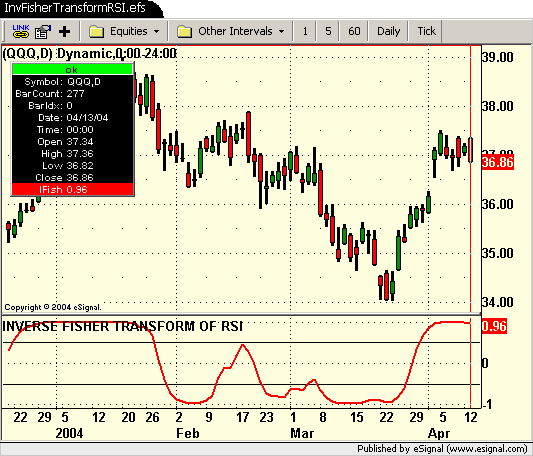# 2004 May: InvFisherTransformRSI.efs

ICE Data Services -

InvFisherTransformRSI.efs

File Name: InvFisherTransformRSI.efs

Description:
Based on The Inverse Fisher Transform by John Ehlers. This article appeared in the May 2004 issue of Stock & Commodities.

Formula Parameters:

NA

Notes:
The related article is copyrighted material. If you are not a subscriber of Stocks & Commodities, please visit www.traders.com.
The May 2004 EFS formulas were provided by TS Support.

InvFisherTransformRSI.efsEFS Code:

```// Magazine:	Technical Analysis of Stocks & Commodities, May 2004
// Article:		The Inverse Fisher Transform by John F. Ehlers
// Study:		INVERSE FISHER TRANSFORM OF RSI
// Provided By:	TS Support, LLC for eSignal

var RSI = null;

function preMain(){
setStudyTitle("INVERSE FISHER TRANSFORM OF RSI");
setCursorLabelName("IFish",0);
setDefaultBarFgColor(Color.red,0);
setDefaultBarThickness(2);
//setComputeOnClose();
}

function main(){
wmaLength = 9;
rsiLength = 5;
if (RSI == null) RSI = new RSIStudy(rsiLength,"close");
var IFish = 0, WtdSum = 0 ;

Value1 = .1 * (RSI.getValue(RSIStudy.RSI) - 50);

for(i = 0; i < wmaLength; i++)
WtdSum += (wmaLength - i) * (.1 * (RSI.getValue(RSIStudy.RSI,-i) - 50)) ;
CumWt = (wmaLength + 1 ) * wmaLength * .5 ;
Value2 = WAverage = WtdSum / CumWt ;

IFish = (Math.exp(2 * Value2) - 1) / (Math.exp(2 * Value2) + 1);

return IFish;
}```